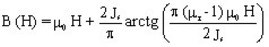# Isotropic / anisotropic soft magnetic material: analytic saturation curve

## Presentation

This model defines a nonlinear B(H) dependence for an isotropic material, taking the saturation into consideration.

## Mathematical model

This model is comprised of a straight line and an arc tangent curve .

The corresponding mathematical formula is written as:where:

• μ0 is the permeability of vacuum, μ0 = 4 π 10-7 H/m
• μr is the initial relative permeability of the material (at the origin)
• Js is the magnetic polarization at saturation T

The shape of this B(H) model is given in the figure below.## Anisotropic material

For an anisotropic material, this linear model consists of a group of three straight lines.

This model is taken into account with the following conditions:

• 2D applications (2D plane domain): Magneto Static / Transient magnetic
• regions: magnetic non conducting, solid conductor